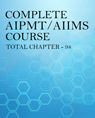Click to Chat

1800-1023-196

+91-120-4616500

CART 0

• 0

MY CART (5)

Use Coupon: CART20 and get 20% off on all online Study Material

ITEM
DETAILS
MRP
DISCOUNT
FINAL PRICE
Total Price: Rs.

There are no items in this cart.
Continue Shopping
`        A stone with weight w is thrown vertically upward into air from ground level with initial speed v0..If a constant force f due to air drag acts on the stone throughout its flight ,the speed of stone just before impact with the ground is what?`
one year ago

```							a)initial energy = 1/2 m v^2 work done by air drag is f h potential energy at maximum height h is mgh ( or the work done by/against gravirty) 1/2mv^2 = fh + mgh h = mv^2 /2(f+mg) or writing interms of W = mg , replace m on top with W/g and mg in the debominator with W you will get the result b) initial kinetic energy = 1/2mv^2 final energy = 1/2mV2^2 where v2 is the final velocity work done by f is 2fh 1/2mv^2 = 1/2mV2^2 + 2fh since there is no change in potential energy 1/2 mv2^2 = 1/2mv^2 -2fh W v2^2 = Wv^2 -4fgh v2^2 = v^2 - 4gf/W * h but we know h = v^2/(2g(1+(f/W))) = Wv^2/(2g(f+W) v2^2 = v^2 - 4gf/W * Wv^2/(2g(f+W)) v2^2 = v^2 - 2f * v^2/((f+W)) = v^2 [(f+W -2f)/(W+f)] = v^2 (W-f)/(W+f) v2 = v^2 √((W-f)/(W+f))
```
one year ago
Think You Can Provide A Better Answer ?

## Other Related Questions on AIPMT

View all Questions »### Course Features

• 731 Video Lectures
• Revision Notes
• Previous Year Papers
• Mind Map
• Study Planner
• NCERT Solutions
• Discussion Forum
• Test paper with Video Solution### Course Features

• 728 Video Lectures
• Revision Notes
• Test paper with Video Solution
• Mind Map
• Study Planner
• NCERT Solutions
• Discussion Forum
• Previous Year Exam Questions